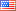##### Modeling of photovoltaic array output current based on actual performance using artificial neural networks
Publication Type
Original research
Authors

This paper presents prediction models for photovoltaic (PV) module's output current. The proposed models are based on empirical, statistical, and artificial neural networks. The adopted artificial neural networks are generalized regression, feed forward, and cascaded forward neural networks. The proposed models have two inputs, namely, solar radiation and ambient temperature, while system's output current is the output. Two years of experimental data for a 1.4 kWp PV system are utilized in this research. These data are recorded every 10 seconds in order to consider the uncertainty of system's output current. Three statistical values are used to evaluate the accuracy of the proposed models, namely, mean absolute percentage error, mean bias error, and root mean square error. A comparison between the proposed models in terms of prediction accuracy is conducted. The results show that the generalized regression neural network based model exceeds the other models. The mean absolute percentage error, root mean square error, and mean bias error of the generalized regression neural network modelare 4.97%, 5.67%, and −1.17%, respectively.

Journal
Title
Journal of Renewable and Sustainable Energy
Publisher
AIP
Publisher Country
United States of AmericaIndexing
Thomson Reuters
Impact Factor
-0.87
Publication Type
Both (Printed and Online)
Volume
7
Year
2015
Pages
053107, 2015